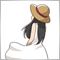# Moving Average of a RSI and RSI161

Hi all,

I am trying to do my own programming in this indicator. I took the default RSI code from MT4 and made some modifications. However, I add in the moving average of the RSI code, the compiler seems ok but when I apply to the chart, there is only an RSI line. The moving average of the RSI is missing. Can anyone let me know what's went wrong? I am using MT4.

```//+------------------------------------------------------------------+
//|                                                          RSI.mq4 |
//|                   Copyright 2005-2014, MetaQuotes Software Corp. |
//|                                              http://www.mql4.com |
//+------------------------------------------------------------------+
#property copyright   "2005-2014, MetaQuotes Software Corp."
#property description "Relative Strength Index with Moving Average"
#property strict

#property indicator_separate_window
#property indicator_minimum    0
#property indicator_maximum    100
#property indicator_buffers    2
#property indicator_color1     DodgerBlue
#property indicator_color2     Red
#property indicator_level1     30.0
#property indicator_level2     70.0
#property indicator_levelcolor clrSilver
#property indicator_levelstyle STYLE_DOT
//--- input parameters
input int InpRSIPeriod=14; // RSI Period
input int InpRSIMAPeriod=9; //Moving Average Period
input ENUM_MA_METHOD InpMAMethod=MODE_SMA;  // Method

//--- buffers
double ExtRSIBuffer[];
double ExtRSIMABuffer[];
double ExtPosBuffer[];
double ExtNegBuffer[];

//+------------------------------------------------------------------+
//| Custom indicator initialization function                         |
//+------------------------------------------------------------------+

int OnInit(void)
{
string short_name;

//--- 2 additional buffers are used for counting.
IndicatorBuffers(4);
SetIndexBuffer(2,ExtPosBuffer);
SetIndexBuffer(3,ExtNegBuffer);

//--- indicator line
SetIndexStyle(0,DRAW_LINE);
SetIndexBuffer(0,ExtRSIBuffer);
SetIndexStyle(1,DRAW_LINE);
SetIndexBuffer(1,ExtRSIMABuffer);

//--- name for DataWindow and indicator subwindow label
short_name="RSI("+string(InpRSIPeriod)+")";
IndicatorShortName(short_name);
SetIndexLabel(0,short_name);

//--- check for input
if(InpRSIPeriod<2 || InpRSIMAPeriod<1)
{
Print("Incorrect value for input variable InpRSIPeriod or InpRSIMAPeriod ");
return(INIT_FAILED);
}

//---
SetIndexDrawBegin(0,InpRSIPeriod);
SetIndexDrawBegin(1,InpRSIMAPeriod);

//--- initialization done
return(INIT_SUCCEEDED);
}
//+------------------------------------------------------------------+
//| Relative Strength Index                                          |
//+------------------------------------------------------------------+

int OnCalculate(const int rates_total,
const int prev_calculated,
const datetime &time[],
const double &open[],
const double &high[],
const double &low[],
const double &close[],
const long &tick_volume[],
const long &volume[],
{
int    i,k,pos;
double diff;

//---
if(Bars<=InpRSIPeriod || InpRSIPeriod<2)
return(0);

//--- counting from 0 to rates_total
ArraySetAsSeries(ExtRSIBuffer,false);
ArraySetAsSeries(ExtRSIMABuffer,false);
ArraySetAsSeries(ExtPosBuffer,false);
ArraySetAsSeries(ExtNegBuffer,false);
ArraySetAsSeries(close,false);

//--- preliminary calculations
pos=prev_calculated-1;
if(pos<=InpRSIPeriod)
{
//--- first RSIPeriod values of the indicator are not calculated
ExtRSIBuffer=0.0;
ExtPosBuffer=0.0;
ExtNegBuffer=0.0;
double sump=0.0;
double sumn=0.0;
for(i=1; i<=InpRSIPeriod; i++)
{
ExtRSIBuffer[i]=0.0;
ExtPosBuffer[i]=0.0;
ExtNegBuffer[i]=0.0;
diff=close[i]-close[i-1];
if(diff>0)
sump+=diff;
else
sumn-=diff;
}

//--- calculate first visible value
ExtPosBuffer[InpRSIPeriod]=sump/InpRSIPeriod;
ExtNegBuffer[InpRSIPeriod]=sumn/InpRSIPeriod;
if(ExtNegBuffer[InpRSIPeriod]!=0.0)
ExtRSIBuffer[InpRSIPeriod]=100.0-(100.0/(1.0+ExtPosBuffer[InpRSIPeriod]/ExtNegBuffer[InpRSIPeriod]));
else
{
if(ExtPosBuffer[InpRSIPeriod]!=0.0)
ExtRSIBuffer[InpRSIPeriod]=100.0;
else
ExtRSIBuffer[InpRSIPeriod]=50.0;
}

//--- prepare the position value for main calculation
pos=InpRSIPeriod+1;
}
//--- the main loop of calculations
for(i=pos; i<rates_total && !IsStopped(); i++)
{
diff=close[i]-close[i-1];
ExtPosBuffer[i]=(ExtPosBuffer[i-1]*(InpRSIPeriod-1)+(diff>0.0?diff:0.0))/InpRSIPeriod;
ExtNegBuffer[i]=(ExtNegBuffer[i-1]*(InpRSIPeriod-1)+(diff<0.0?-diff:0.0))/InpRSIPeriod;
if(ExtNegBuffer[i]!=0.0)
ExtRSIBuffer[i]=100.0-100.0/(1+ExtPosBuffer[i]/ExtNegBuffer[i]);
else
{
if(ExtPosBuffer[i]!=0.0)
ExtRSIBuffer[i]=100.0;
else
ExtRSIBuffer[i]=50.0;
}
}

//--- signal
pos=InpRSIMAPeriod-1;
if(pos+1<prev_calculated)
pos=prev_calculated-2;
else
{
for(i=0; i<pos; i++)
ExtRSIMABuffer[i]=0.0;
}
for(i=pos; i<rates_total && !IsStopped(); i++)
{
double sum=0.0;
for(k=0; k<InpRSIMAPeriod; k++)
sum+=ExtRSIMABuffer[i-k];
ExtRSIMABuffer[i]=sum/InpRSIMAPeriod;
}
//---
return(rates_total);
}
//+------------------------------------------------------------------+
```525

```for(i=pos; i<rates_total && !IsStopped(); i++)
{
double sum=0.0;
for(k=0; k<InpRSIMAPeriod; k++)
sum+=ExtRSIMABuffer[i-k];
ExtRSIMABuffer[i]=sum/InpRSIMAPeriod;
}     ```

Why do you calculate the sum of ExtRSIMABuffer?

You have to put this question in "MQL4" section.161

Why do you calculate the sum of ExtRSIMABuffer?

You have to put this question in "MQL4" section.

If I don't calculate the ExtRSIMABuffer, how does the MT4 plot it?

Where is the MT4 section?525

kentaicm:

If I don't calculate the ExtRSIMABuffer, how does the MT4 plot it?

Where is the MT4 section?

You want to calculate the average of "ExtRSIBuffer", don't you?

It's impossible to get it from the sum of "ExtRSIMABuffer".

MT4 section.161

You want to calculate the average of "ExtRSIBuffer", don't you?

It's impossible to get it from the sum of "ExtRSIMABuffer".

MT4 section.

Yes, but how will you write this indicator?525

kentaicm:
Yes, but how will you write this indicator?
`sum+=ExtRSIBuffer[i-k];`